# How to create a step chart in Excel

Excel
Excel offers many different pre-defined chart types, but for some data, pre-defined charts such as area charts or line charts can look fine but are incorrect.

For example, for the changes in prices, invested amounts, or any discrete data - data changes at some regular or irregular points, but the value remains constant between the points:

• On the column or bar chart, give a correct representation,
• On an area chart or any kind of line or scatter chart, it looks strange: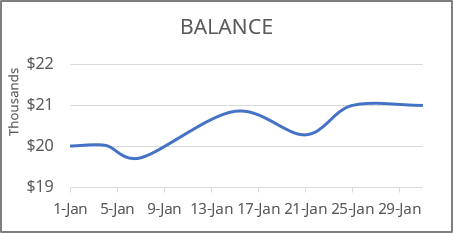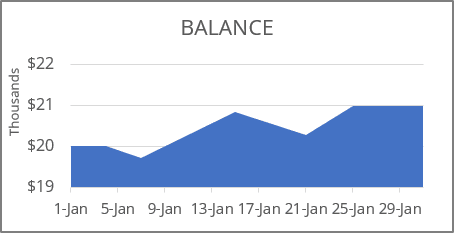Why is this strange? It looks like the data changes every day (month, year). For this example, you seem to deposit or withdraw some amount daily.

To display discrete data, you can create a step chart, also known as a stepped line chart, where values change discretely at specific points.

To create a correct step chart in Excel, you need to make one simple additional step - add the intermediate data:

For example, if the data for the chart looks like this: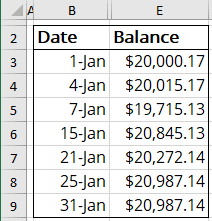For a step chart, you will need two values for the same date (for example, for the January 4th):

• The initial value should be equal to the preceding date value.

For this example, = \$20,000.17 is the value on January 1st.

• The end value should be equal to the current value.

For this example, = \$20,015.17.

For this example: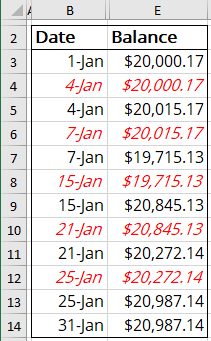After adding the data, just create a line chart or an area chart: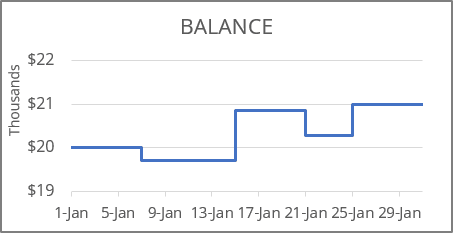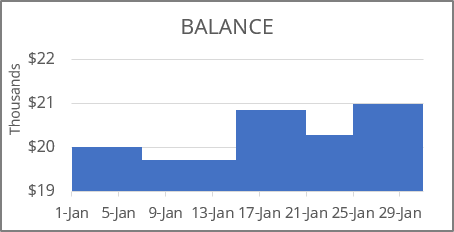See also this tip in French: Comment créer un graphique en étapes dans Excel.